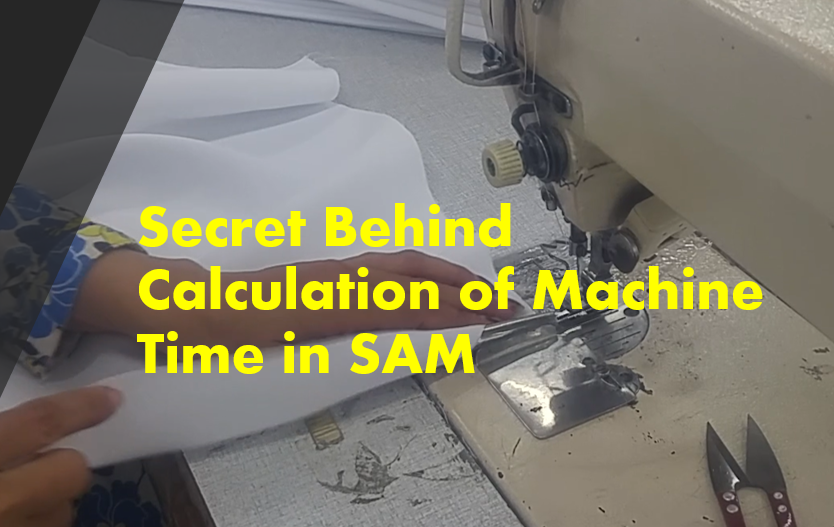# Secret Behind Calculation of Machine Time in SAMStandard Allowed Minute (SAM) of an operation is the sum of 3 different parameter, i.e. machine time, material handling (with personal allowances) time and bundle time. Material handling and bundle time is calculated by motion analysis.

Do you know how the machine time is being calculated?

A standard formula is used to calculate machine time. In this article it has been explained in details. In this article you will get information on how machine time is calculated for SAM.

Machine time consist of 5 parameters as the following
1. Straight machine time (A)
2. Number of stops/starts in a seam (B)
3. Stopping Accuracy (C)
4. Difficulty of material handling (D)
5. Machine allowance (E)
1. Straight machine Time (A):
Straight machine time is explained as the time needed in sewing a seam without considering accurate stop at the end of the seam, material handling and machine allowance. To calculate straight machine time you need Machine RPM, SPI and Seam length.

Formula: Machine Time (A) = SPI X Seam Length/RPM

To represent machine time in TMU (Time measuring unit), above value (A) is divided by 0.0006

2. Number of stops/starts in a seam (B):
Every time operator stops machine to align, realign panels or for other motions, a fixed time is added to machine time. Normally it is 17 TMU (for stop 8.5 TMU and for start 8.5 TMU). If machine is stopped twice in a single seam then 2 times 17 will be added to machine time. Let’s represent number stop/start in a operation as ‘B’.

3. Stopping Accuracy (C):
At the time of sewing a seam how would you like to stop the machine? Machine stop could be a precise stop or normal stop. For example, during stitching of a pointed collar, machine must be stopped at the collar point accurately otherwise you do not get sharp corner. Stopping accuracy can be categorized in different level of accuracy. A differential TMU value is added to machine time according to stopping accuracy. Let’s represent it as ‘C’.

4. Difficulty of handling (D):
At the time of sewing garments operator need to hold and guide fabric panels to get stitches on the desired line. Difficulty of handling garment components depends on no. of fabric plies to be sewn, types of fabric is used, shape and type of seam. It is obvious that sewing a straight line is easier than sewing a curvy line. Handling multiple plies is more difficult than single ply. According to the material handling difficulty level the sum of above three (A+B+C) is multiplied with difficulty factor. Factors used such as 1.1, 1.2, 1.3 etc. Let’s represent it as ‘D’.

5. Machine Allowance (E): A certain percentage of allowance is added to machine time for machine allowance. Machine allowance is added due to certain non-avoidable tasks such as threading of machine, changing of bobbin and machine breakdown needed to perform during sewing. There is pre-defined allowance percentage for machine allowances. Machine Allowance is added to the result of above four parameters.

Now to calculate the Final machine time following formula is used

= [{(straight machine Time + No. of start/stops + stopping accuracy) X difficulty factor in Material Handling} + {(A+17B+C) X D} X Machine allowance %]

= [{(A+17B+C) X D} + {(A+17B+C) X D} X E%]

= (1+E %) X {(A+17B+C) X D}

Read this book to know more about how to calculate machine time Sewing-Performance & Methods-Analysis. Revised & Enlarged 2nd Edition.authored by D. G. Stohlman.